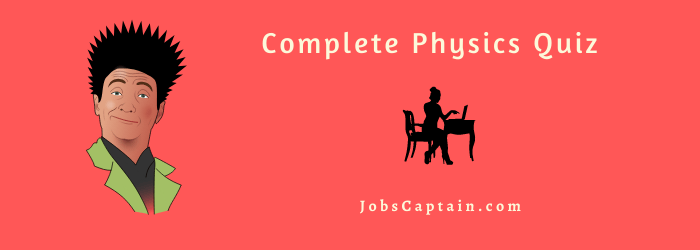# Physics Quiz Questions## Physics Quiz Questions With Answers

Question 1: Which one is supplementary unit?

(A) second

(B) Ampere

(C) Candella

Question 2: Parsec is unit of……

(A) Distance

(B) Velocity

(C) Time

(D) Plane angle

(A) Distance

Question 3: Which one is unit of Intensity of an electric field?

(A) Vm

(B) NC

(C) Vm-1

(D) As

(C) Vm-1

Question 4: Which one is not a unit of time?

(A) second

(B) hour

(C) year

(D) lightyear

(D) lightyear

Question 5: Which one is not a physical quantity?

(A) Kelvin

(B) Candella

(C) Volt

(D) All

(D) All

Question 6: Which physical quantity having the same unit in all the unit systems?

(A) Length

(B) Time

(C) Mass

(D) Work

(B) Time

Question 7: dyne g.1 is a unit of which physical quantity?

(A) Velocity

(B) mass

(C) Force

(D) Acceleration

(D) Acceleration

Question 8: Which physical quantity from given below is dimensionless?

(A) Angle

(B) Stress

(C) density

(D) Latent heat

(A) Angle

Question 9: The average distance between sun and earth is called _____.

(A) 1 Parsec

(B) 1 lightyear

(C) 1 AU

(D) 1A°

(C) 1 AU

Question 10: Which unit is different than other units?

(A) Ws

(B) KWh

(C) Js

(D) eV

(C) Js

Question 11: If the units for mass, length and time become double, then unit of angular momentum becomes ____.

(A) Doubles

(B) Three times

(C) Four times

(D) Eight times

(C) Four times

Question 12:  Ns is a unit of which physical quantity?

(A) velocity

(B)Angular momentum

(C) Linear momentum

(D) work

(C) Linear momentum

Question 13: The volume and area of the surface are equal for a given cube. Then the surface area = ____ unit.

(A) 36

(B) 216

(C) 144

(D) 1000

(B) 216

Question 14: Nm-2 is not a unit of physical quantity given below?

(A) Pressure

(B) Stress

(C) Bulk modulus

(D) Strain

(D) Strain

Question 15: If the unit of length and force increases to four times, the unit of energy _________.

(A) Increases to 8 times

(B) Increases to 16 times

(C) Decreases to 8 times

(D) Decreases to 16 times

(B) Increases to 16 times

Question 16: If the unit of length and time are taken as km and hr, what is the value of g in km h-1.

(A) 980

(B) 9800

(C) 1,27,008

(D) 12,700

(C) 1,27,008

Question 17: For a wire, mass = (0.3 ± 0.003) g radius = (0.5 ± 0.005) mm and length = (6 ± 0.06) cm, percentage error in density is _____ .

(A) 1 %

(B) 2 %

(C) 3 %

(D) 4 %

(D) 4 %

Question 18: The periodic time of second pendulum is 2.0 s and the mean absolute error in its measurement is 0.01s, then value of periodic time with error is _____.

(A) 2.0 ± 0.10 s

(B) 2.0 ± 0.05 s

(C) 2.0 ± 0.02 s

(D) 2.0 ± 0.01 s

(D) 2.0 ± 0.01 s

Question 19: How many significant digits in 0.0250?

(A) 1

(B) 2

(C) 3

(D) 4

(C) 3

Question 20: 1.875 + 2.41 = _____ (by considering significant digits)

(A) 4.3

(B) 4.28

(C) 4.285

(D) 4.29

(B) 4.28

Question 21: Significant digits in 0.0007 are …

(A) 1

(B) 2

(C) 3

(D) 4

(A) 1

Question 22: When 1.71 N is Subtracted from 3.75 N the result in significant figures is ______.

(A) 2 N

(B) 2.0 N

(C) 2.04 N

(D) 2.000 N

(C) 2.04 N

Question 23 : Thickness of plate measured by micrometer having least count 0.01mm is 1.03 mm. What is the percentage error in the measurement of the thickness of plate?

(A) 0.7 %

(B) 0.97 %

(C) 1 %

(D) 1.2 %

(B) 0.97 %

Question 24: 9.15 + 3.8 = _____ (by considering significant digits).

(A) 13

(B) 13.0

(C) 13.00

(D) 13.000